Courses

# Ratio and Proportion - Important Formulas, Quantitative Aptitude Quant Notes | EduRev

## UPSC : Ratio and Proportion - Important Formulas, Quantitative Aptitude Quant Notes | EduRev

The document Ratio and Proportion - Important Formulas, Quantitative Aptitude Quant Notes | EduRev is a part of the UPSC Course SSC CGL Tier 2 - Study Material, Online Tests, Previous Year.
All you need of UPSC at this link: UPSC

Ratio & Proportion

Ratio and Proportion are explained majorly based on fractions. When a fraction is represented in the form of a:b, then it is a ratio whereas a proportion states that two ratios are equal.

• Here, a and b are any two integers.
• The ratio and proportion are the two important concepts, and it is the foundation to understand the various concepts in mathematics as well as in science.
•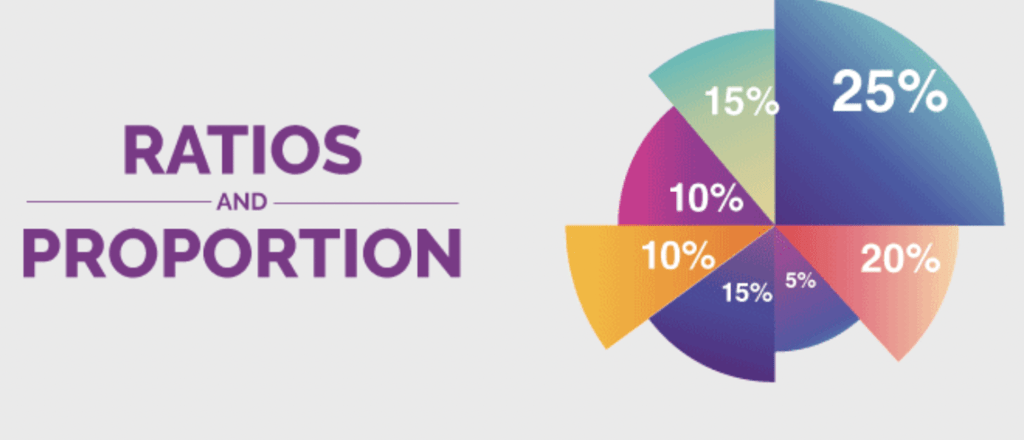Ratio and proportions are said to be faces of the same coin. When two ratios are equal in value, then they are said to be in proportion. In simple words, it compares two ratios.
• Proportions are denoted by the symbol  ‘::’ or ‘=’.

What is a Ratio?

The ratio is the comparison between similar types of quantities, it is an abstract quantity and does not have any units.

• The ratio of two quantities a and b in the same units is the fraction a/b and we write it as a: b.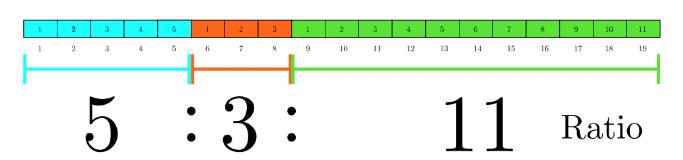• In the ratio a: b, we call the first term or antecedent and b, the second term or consequent.
Example: The ratio 5 : 9 represents 5/9 with antecedent = 5, consequent = 9.

Note: The multiplication or division of each term of a ratio by the same non-zero number does not affect the ratio. Example: 4 : 5 = 8 : 10 = 12 : 15. Also, 4 : 6 = 2 : 3

Key Points to Remember:

• The ratio should exist between the quantities of the same kind.
• While comparing two things, the units should be similar.
• There should be significant order of terms.
• The comparison of two ratios can be performed, if the ratios are equivalent like the fractions.

Try yourself:A and B together have Rs. 1210. If (4 / 15) of A's amount is equal to (2 / 5) of B's amount, how much amount does B have?

What is a Proportion?

The equality of two ratios is called proportion i.e. If a/b = c/d, then a, b, c, d are said to be in proportion.

• If a: b = c : d, we write a: b:: c : d and saying that a, b, c, d are in proportion.
• Here a and d are called Extremes, while b and c are called Mean terms.

Product of means = Product of extremes

Thus, a : b :: c : d ⇔ (b x c) = (a x d)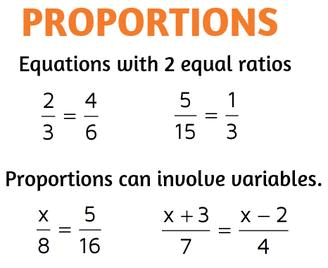Try yourself:Two numbers are respectively 20% and 50% more than a third number. The ratio of the two numbers is:

• Fourth Proportional
If a: b = c : d, then d is called the fourth proportional to a, b, c.
• Third Proportional
If a : b = c : d, then c is called the third proportion to a and b.
• Mean Proportional:
Mean proportional between a and b is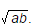Comparison of Ratios

We say that (a : b) > (c : d) ⇔ a/b > c/d.

Compounded Ratio:

The compounded ratio of the ratios:
(a : b), (c : d), (e : f) is (ace : bdf)

Try yourself:In a mixture of 60 litres, the ratio of milk and water 2 : 1. If this ratio is to be 1 : 2, then the quantity of water to be further added is:

Duplicate Ratios

• The duplicate ratio of (a : b) is (a2 : b2)
• Sub - duplicate ratio of (a : b) is (√a : √b)
• Triplicate ratio of (a : b) is (a: b3)
• Sub - triplicate ratio of (a : b) is (a1/3: b1/3)
If a/b = c/d, then a + b/a - b = c + d/c - d [componendo and dividendo]

Variations

• We say that x is directly proportional to y if x = ky for some constant k and we write x ∝ y.
• We say that x is inversely proportional to y, if xy = k for some constant K and we write, x ∝ 1/y.

Types of Variation:

(i) Direct Variation: If A is in direct variation with B, then an increase or decrease in A will lead to a proportionate increase or decrease in B.

• ∝ B
• A = KB

(ii) Indirect Variation: If A is in inverse variation with B, then an increase in A will lead to a Proportionate decrease in B and vice versa.

• ∝ 1/B
• A = K/B

Try yourself:If ‘x’ and ‘y’ are in a direct proportion then which of the following is correct?

(iii) Joint Variation

• Let us consider the area of a triangle, which is dependent on both, the height as well as the base of the rectangle.
• When both the dimension of the triangle changes, then the area also changes.
When the area of the triangle varies with the change in the base of the triangle.

∝ b

• When the area of the triangle varies with the change in the height of the triangle.

∝ h

• This is called the joint variation of the area of the triangle with respect to its base and height.

∝ 1/2 x b.h

Simple Method

The LCM process gets very cumbersome when we have to find the ratio out of multiple ratios.
We have the following simple method for that for a chain of ratios of any length.

Suppose you have the ratio train as follows

A : B = 1 : 2

⇨ B : C = 2 : 3

⇨ C : D = 5 : 6

⇨ D : E = 7 : 8

If we were to find A : B : C : D : E, then the LCM method would have taken quite a long time which is infeasible in examinations of limited hours.

The short cut is as follows:

A : B : C : D : E can be written directly as:

⇨ 1 × 2 × 5 × 7 : 2 × 2 × 5 × 7 : 2 × 3 × 5 × 7 : 2 × 3 × 6 × 7 : 2 × 3 × 6 × 8

⇨ 70 : 140 : 210 : 252 : 288

The thought algorithm for this case goes as:
To get the combined ratio of A : B : C : D : E, from A : B, B : C, C : D, and D : E
In the combined ratio of A : B : C : D : E.

• A will correspond to the product of all numerators (1 × 2 × 5 × 7).
• B will take the first denominator and the last 3 numerators (2 × 2 × 5 × 7).
• C, on the other hand, takes the first two denominators and the last 2 numerators (2 × 3 × 5 × 7).
• D takes the first 3 denominators and the last numerator (2 × 3 × 6 × 7) and E take all the four denominators (2 × 3 × 6 × 8).

Product of Proportions

If a:b = c:d is a proportion, then:

• Product of extremes = product of means i.e., ad = bc
• Denominator addition/subtraction: a:a+b = c:c+d and a:a-b = c:c-d
• a, b, c, d,.... are in continued proportion means, a:b = b:c = c:d = ....
• a:b = b:c then b is called mean proportional and 2b = ac
• The third proportional of two numbers, a and b, is c, such that, a:b = b:c.
d is fourth proportional to numbers a, b, c if a:b = c:d.

Variations

• If a ∝ b, provided c is constant and a ∝ c, provided b is constant, then a ∝ b ∝ c,  if all three of them are varying.
• If A and B are in a business for the same time, then Profit distribution ∝ investment (Time is constant).
• If A and B are in a business with the same investment, then:

Profit distribution ∝ Time of investment (Investment is constant)

Profit Distribution  ∝ Investment × Time

Ratio and Proportion Tricks

• If u/v = x/y, then uy = vx
• If u/v = x/y, then u/x = v/y
• If u/v = x/y, then v/u = y/x
• If u/v = x/y, then (u+v)/v = (x+y)/y
• If u/v = x/y, then (u-v)/v = (x-y)/y
• If u/v = x/y, then (u+v)/ (u-v) = (x+y)/(x-y), which is known as Componendo-Dividendo Rule
• If a/(b+c) = b/(c+a) = c/(a+b) and a+b+ c ≠0, then a =b = c

Solved Questions

Q.1. In a library, the ratio of number of story books to that of non-story books was 4:3 and total number of story books was 1248. When some more story books were bought, the ratio became 5:3. Find the number of story books bought.

a. 312
b. 321
c. 936
d. 1560

Explanation: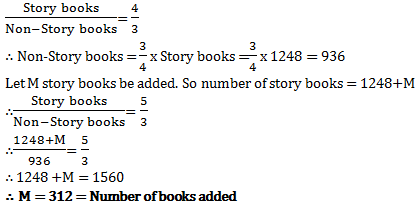Q.2. Rs. 8400 is divided among A, B, C and D in such a way that the shares of A and B, B and C, and C and D are in the ratios of 2:3, 4:5, and 6:7 respectively. The share of A is

a. Rs. 1280
b. Rs. 8400
c. Rs. 8210
d. Rs. 1320

Explanation: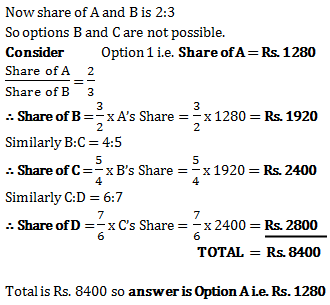Q.3. The ratio of the present age of father to that of son is 7:2. After 10 years their ages will be in the ratio of 9:4. The present ages of the father is

a. 35 years
b. 40 years
c. 30 years
d. 25 years

Explanation: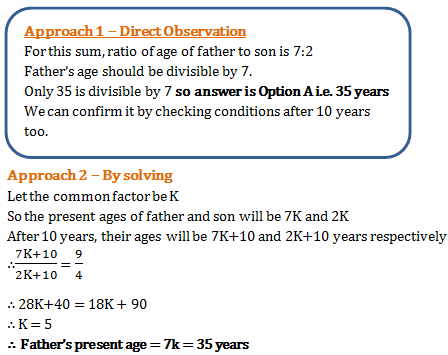Q.4. Ajay and Raj together have Rs. 1050. On taking Rs. 150 from Ajay, Ajay will have same amount as what Raj had earlier. Find the ratio of amounts with Ajay and Raj initially.

a. 3:4
b. 7:1
c. 1:3
d. 4:3

Explanation: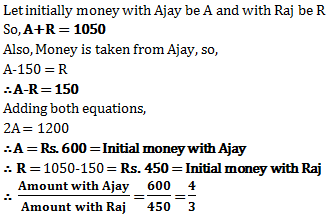Q.5. Price of each article of type P, Q, and R is Rs. 300, Rs. 180 and Rs. 120 respectively. Suresh buys articles of each type in the ratio 3:2:3 in Rs. 6480. How many articles of type Q did he purchase?

a. 8

b. 14
c. 20
d. None of the above

Explanation: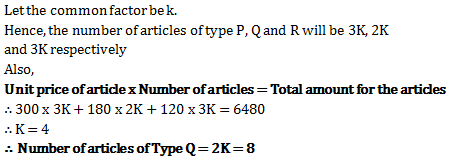Offer running on EduRev: Apply code STAYHOME200 to get INR 200 off on our premium plan EduRev Infinity!

## SSC CGL Tier 2 - Study Material, Online Tests, Previous Year

45 videos|26 docs|42 tests

,

,

,

,

,

,

,

,

,

,

,

,

,

,

,

,

,

,

,

,

,

,

,

,

;